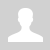Python. Functions. Variable scope. Local and global variables. (en)

11.3.1.3 define the scope of variables

# Python. Functions. Variable scope. Local and global variables.

A local variable is a variable declared inside a function and allows its value to be used only in the body of the function. The value of such a variable exists as long as the function is executed.

Example,

```def name_of_function():     x = 10 # local variable     print(x) # output 10 name_of_function() print(x) # NameError: name 'x' is not defined```

A global variable is a variable whose scope is the entire program unless redefined.

Example,

```x = 20 # global variable def name_of_function():     x = 10 # local variable     print(x) # output 10 name_of_function() print(x) # output 20```

A global variable can also be declared in a function using "global".

Example,

```x = 20 # global variable def name_of_function():     global x # global variable     x = 10      print(x) # output 10 name_of_function() print(x) # output 10```

Questions:

Exercises: